﻿ Mass energy equivalence stock illustration. Illustration of university - 41702285

# Mass energy equivalence.

Royalty-Free Illustration## Golden mass energy equivalence symbol on black background

More similar stock illustrations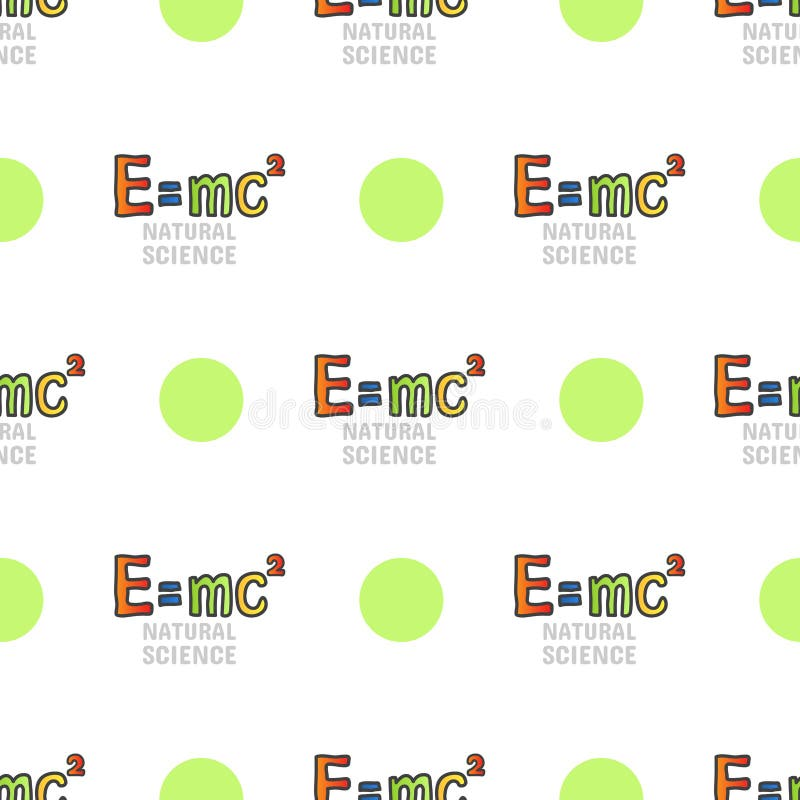Back to school seamless pattern, mass energy equivalenceMass Energy Equivalence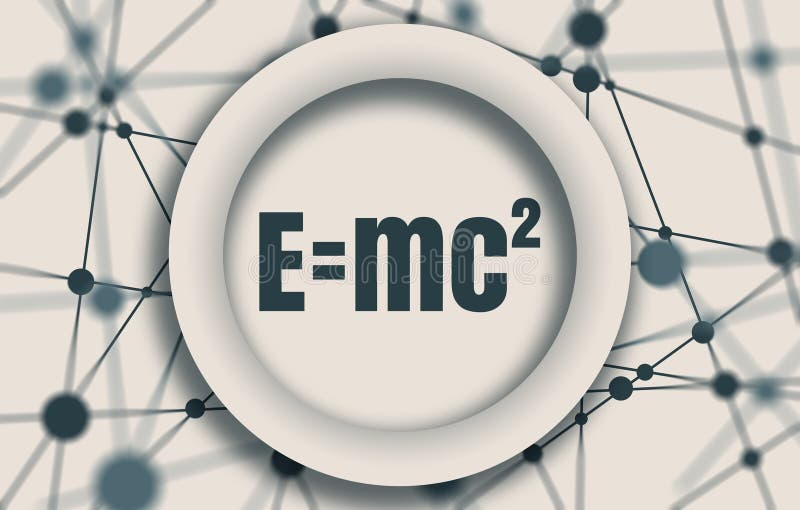Physical formula of mass and energy equivalence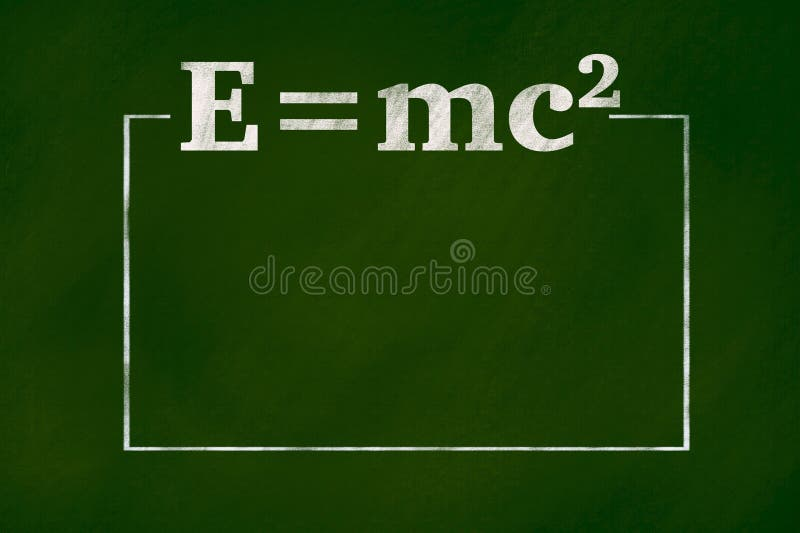mass-energy equivalent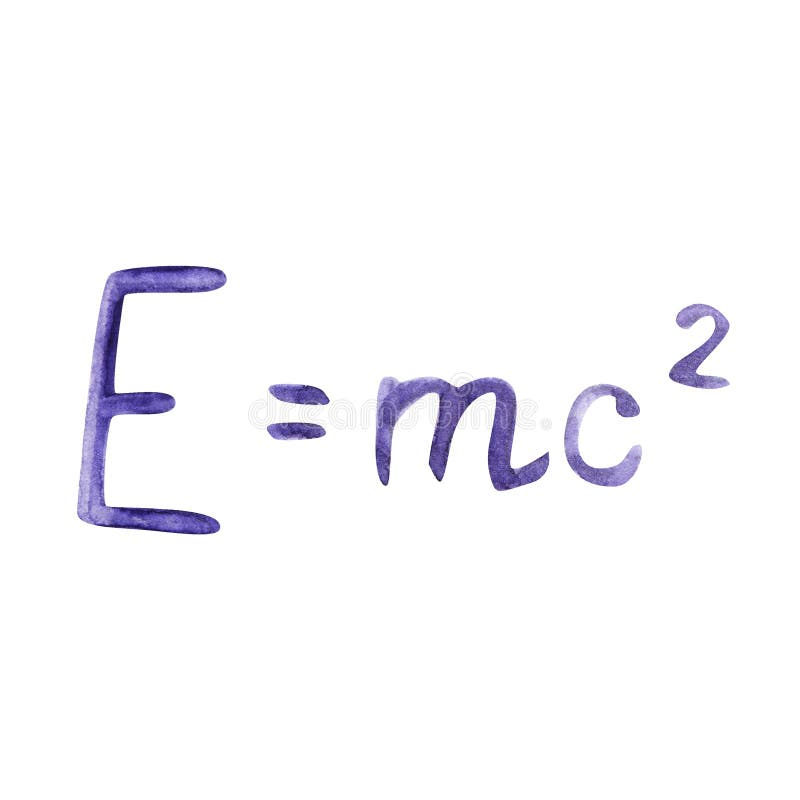Watercolor illustration drawn with formula. The famous formula E mc2. Formula expressing the equivalence of mass and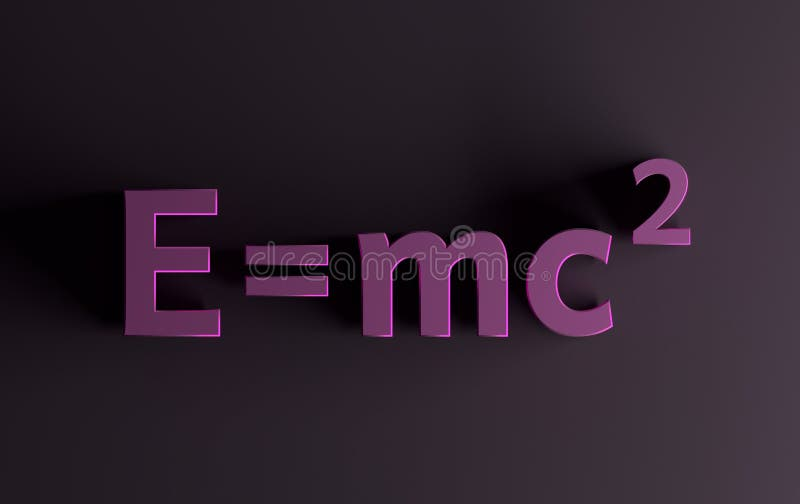Mass energy formula in pink colors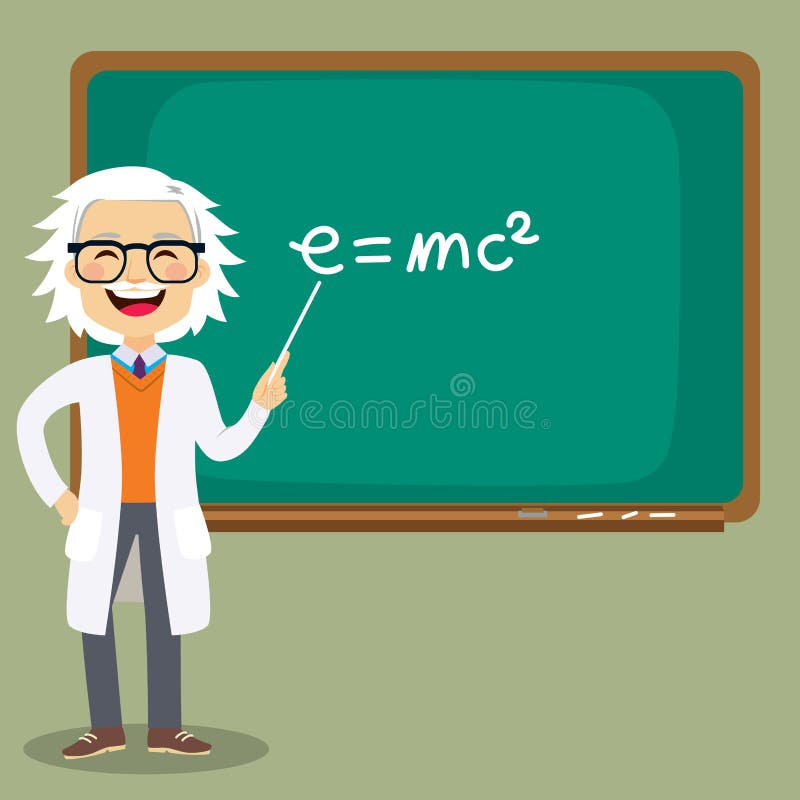Senior Science Teacher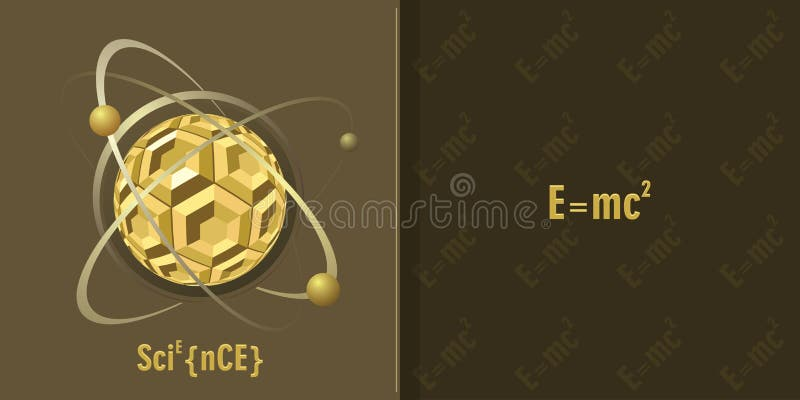Science background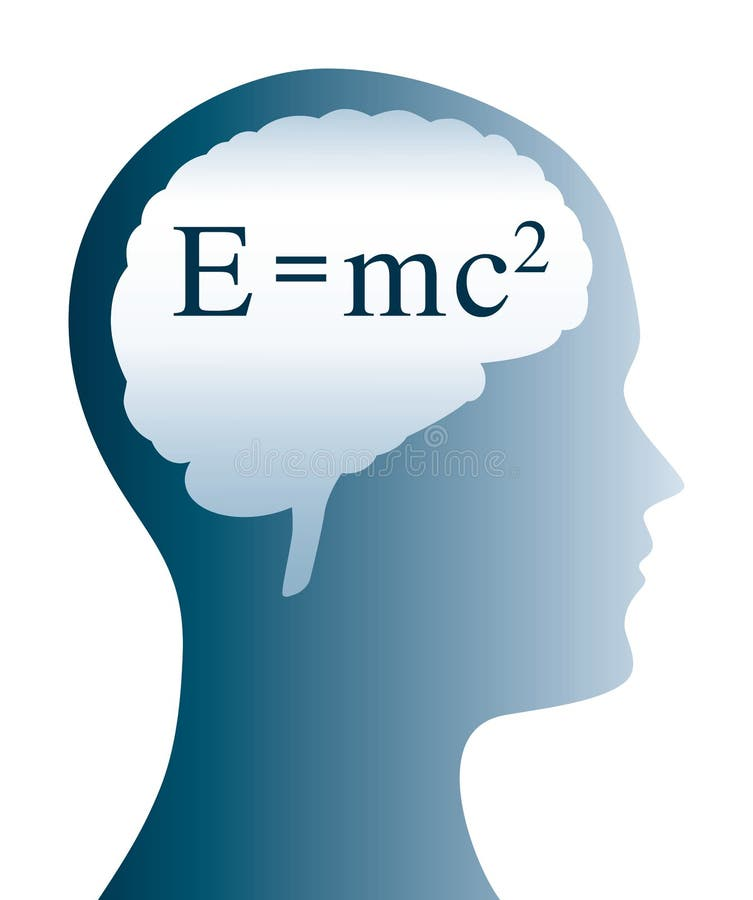E=mc2 Einstein formula in brain and head silhouette
More stock photos from Sgame's portfolio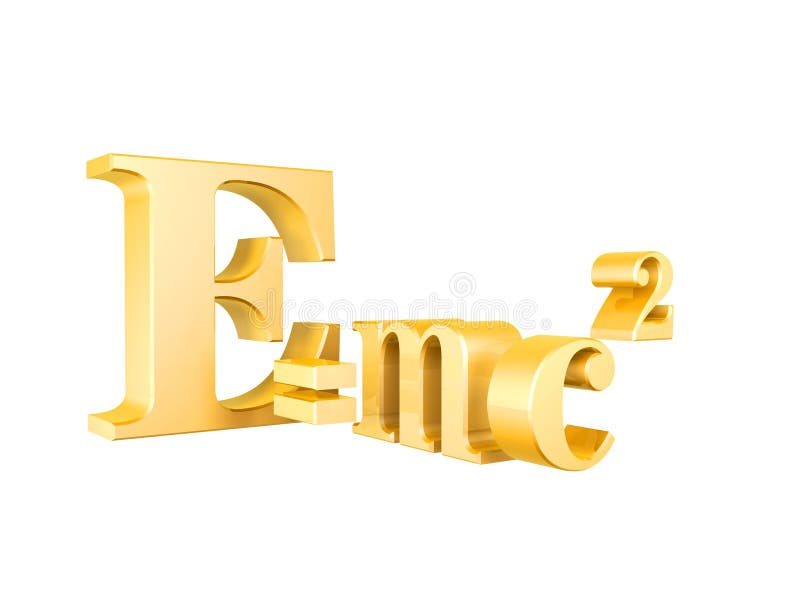Mass energy equivalence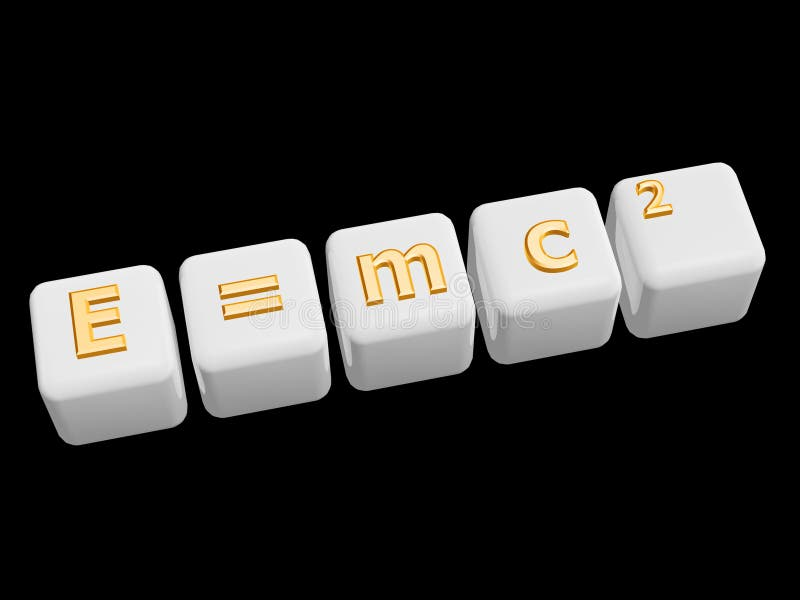Mass energy equivalence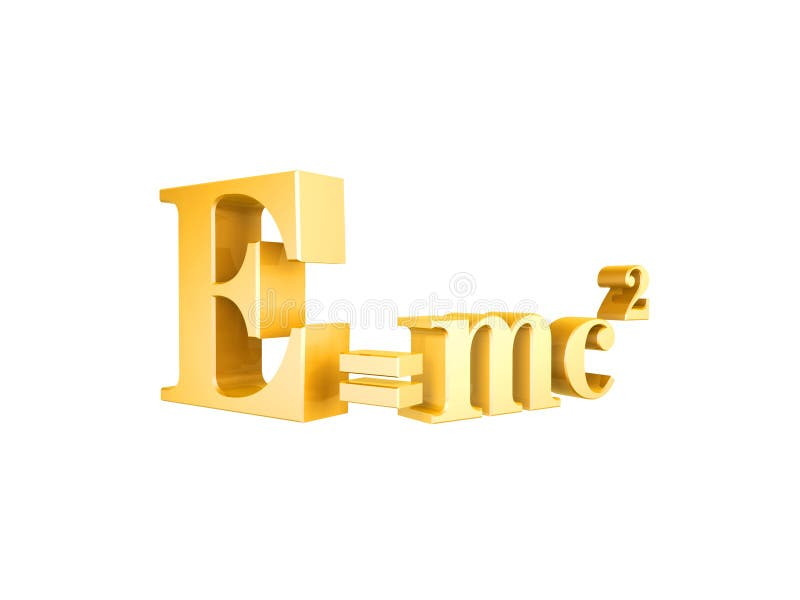Mass energy equivalence